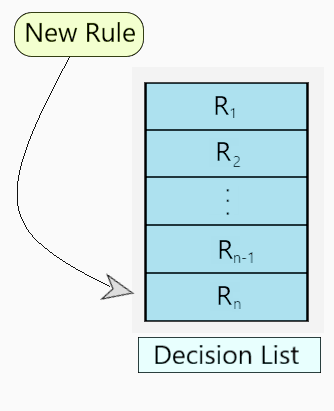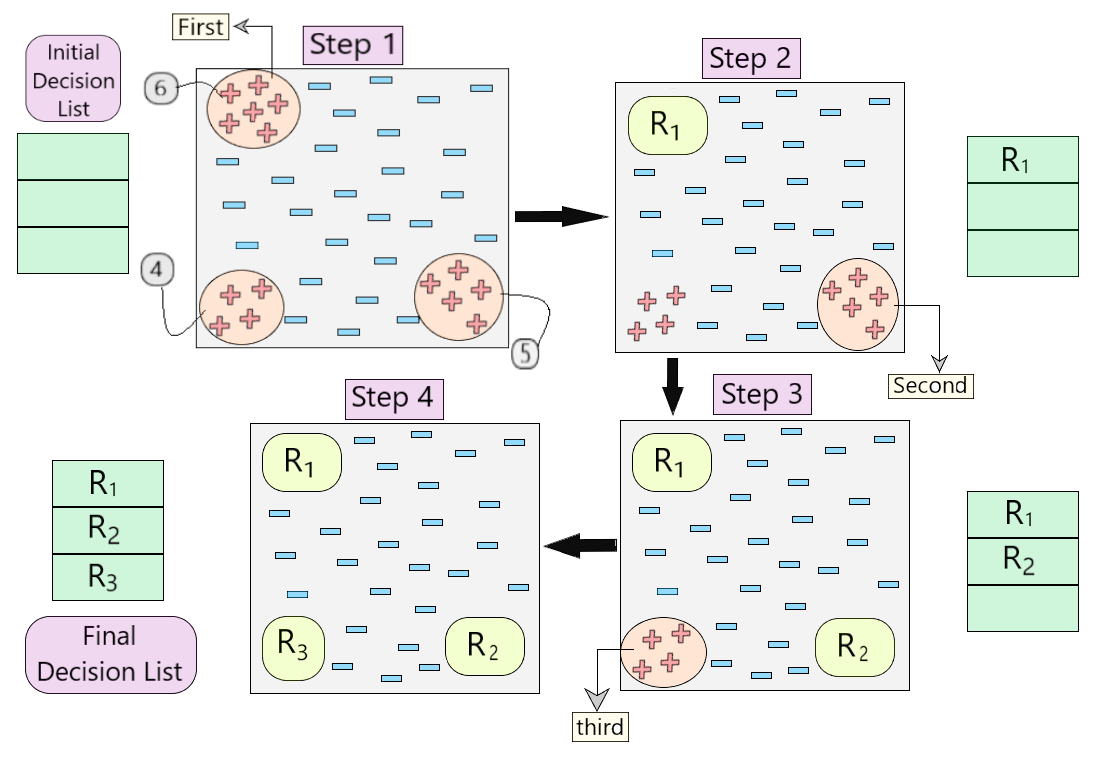Open in App
Not now

# Sequential Covering Algorithm

• Last Updated : 26 Nov, 2020

Prerequisites: Learn-One-Rule Algorithm

Sequential Covering is a popular algorithm based on Rule-Based Classification used for learning a disjunctive set of rules. The basic idea here is to learn one rule, remove the data that it covers, then repeat the same process. In this process, In this way, it covers all the rules involved with it in a sequential manner during the training phase.

Algorithm Involved:

Sequential_covering (Target_attribute, Attributes, Examples, Threshold):
Learned_rules = {}
Rule = Learn-One-Rule(Target_attribute, Attributes, Examples)

while Performance(Rule, Examples) > Threshold :
Learned_rules = Learned_rules + Rule
Examples = Examples - {examples correctly classified by Rule}
Rule = Learn-One-Rule(Target_attribute, Attributes, Examples)

Learned_rules = sort Learned_rules according to performance over Examples
return Learned_rules


The Sequential Learning algorithm takes care of to some extent, the low coverage problem in the Learn-One-Rule algorithm covering all the rules in a sequential manner.

Working on the Algorithm:

The algorithm involves a set of ‘ordered rules’ or ‘list of decisions’ to be made.

Step 1 – create an empty decision list, ‘R’.
Step 2 – ‘Learn-One-Rule’ Algorithm
It extracts the best rule for a particular class ‘y’, where a rule is defined as: (Fig.2)

General Form of RuleIn the beginning,
Step 2.a – if all training examples ∈ class ‘y’, then it’s classified as positive example.
Step 2.b – else if all training examples ∉ class ‘y’, then it’s classified as negative example.

Step 3 – The rule becomes ‘desirable’ when it covers a majority of the positive examples.
Step 4 – When this rule is obtained, delete all the training data associated with that rule.
(i.e. when the rule is applied to the dataset, it covers most of the training data, and has to be removed)

Step 5 – The new rule is added to the bottom of decision list, ‘R’. (Fig.3)Fig 3 : Decision List ‘R’

Below, is a visual representation describing the working of the algorithm.Fig 4: Visual Representation of working of the algorithm

• Let us understand step by step how the algorithm is working in the example shown in Fig.4.
• First, we created an empty decision list. During Step 1, we see that there are three sets of positive examples present in the dataset. So, as per the algorithm, we consider the one with maximum no of positive example. (6, as shown in Step 1 of Fig 4)
• Once we cover these 6 positive examples, we get our first rule R1, which is then pushed into the decision list and those positive examples are removed from the dataset. (as shown in Step 2 of Fig 4)
• Now, we take the next majority of positive examples (5, as shown in Step 2 of Fig 4) and follow the same process until we get rule R2. (Same for R3)
• In the end, we obtain our final decision list with all the desirable rules.

Sequential Learning is a powerful algorithm for generating rule-based classifiers in Machine Learning. It uses ‘Learn-One-Rule’ algorithm as its base to learn a sequence of disjunctive rules. For doubts/queries regarding the algorithm, comment below.

My Personal Notes arrow_drop_up
Related Articles